# Sampling distribution 1 Sampling distribution 2 Sampling distribution

• Slides: 80Sampling distribution 1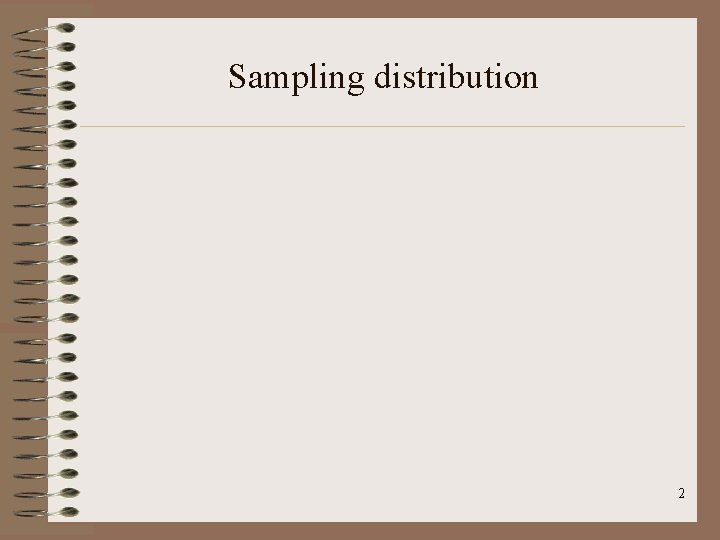Sampling distribution 2Sampling distribution 3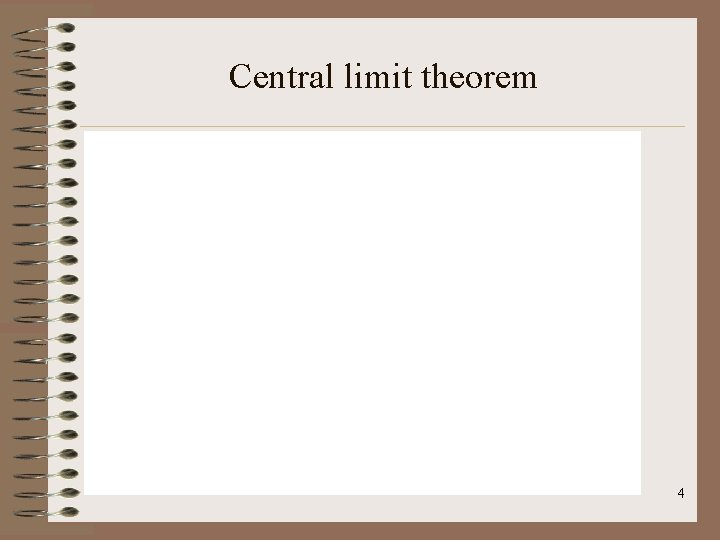Central limit theorem 4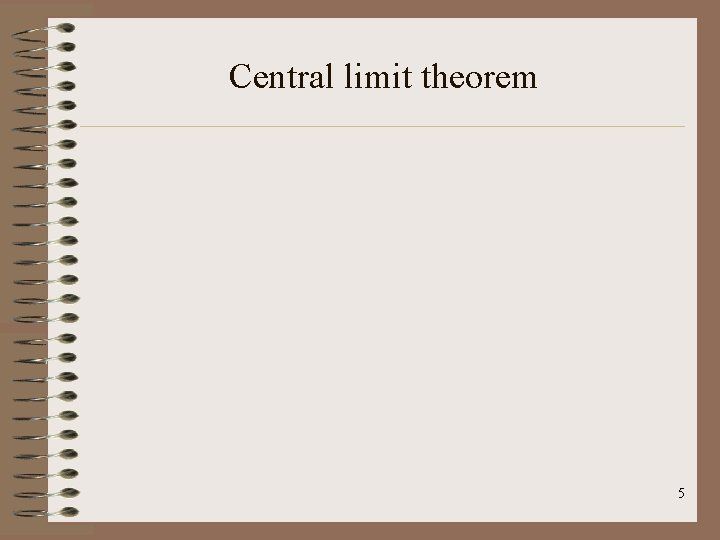Central limit theorem 5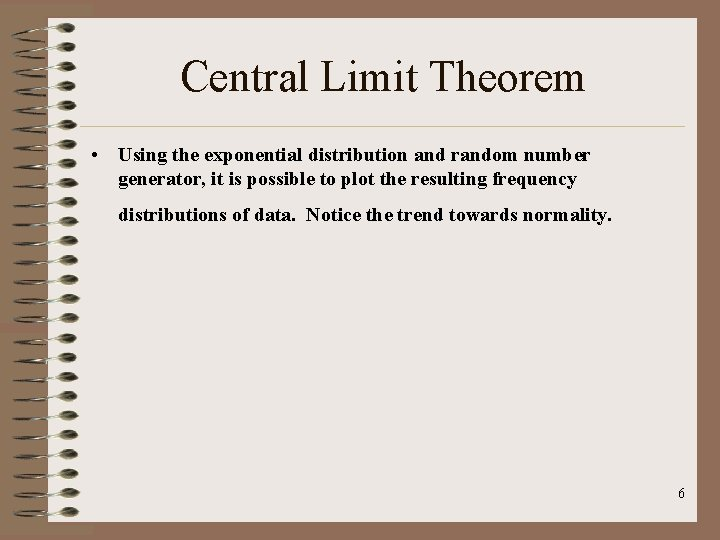Central Limit Theorem • Using the exponential distribution and random number generator, it is possible to plot the resulting frequency distributions of data. Notice the trend towards normality. 6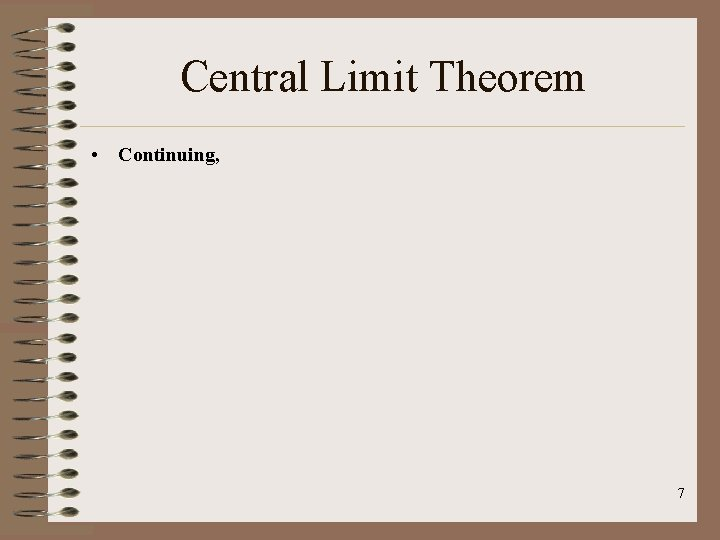Central Limit Theorem • Continuing, 7T-Distribution 8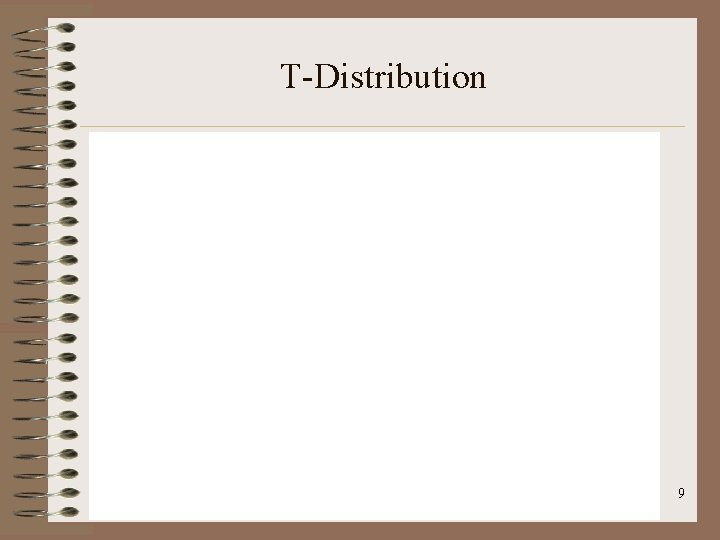T-Distribution 9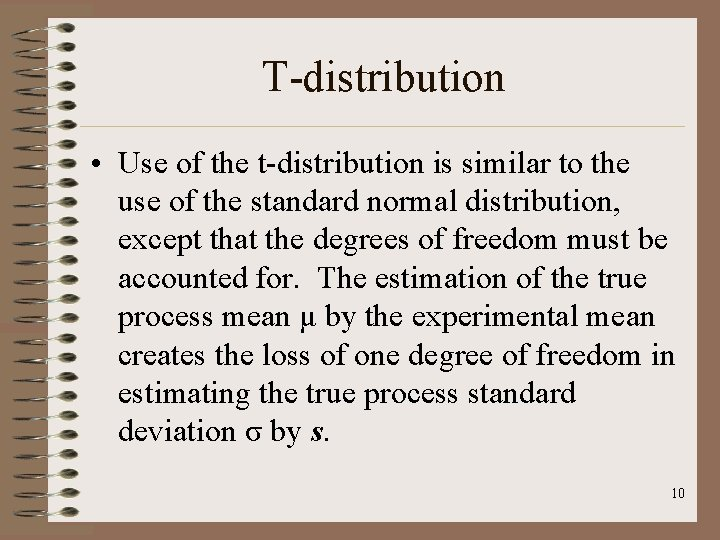T-distribution • Use of the t-distribution is similar to the use of the standard normal distribution, except that the degrees of freedom must be accounted for. The estimation of the true process mean μ by the experimental mean creates the loss of one degree of freedom in estimating the true process standard deviation σ by s. 10Confidence Interval 11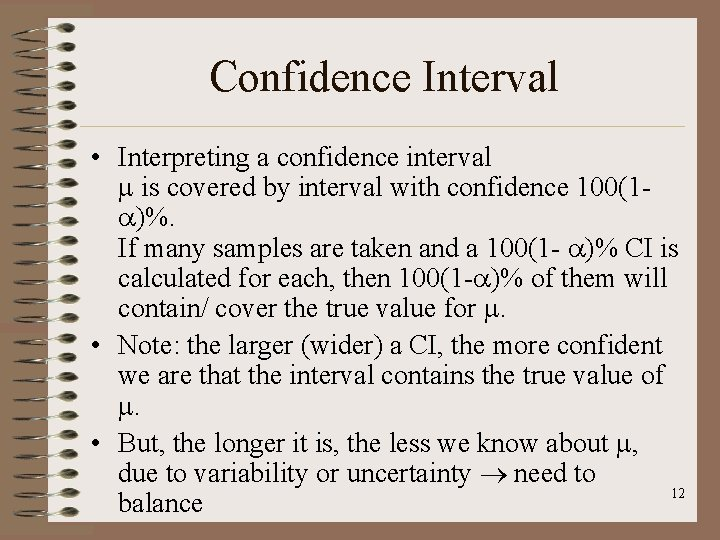Confidence Interval • Interpreting a confidence interval is covered by interval with confidence 100(1 )%. If many samples are taken and a 100(1 - )% CI is calculated for each, then 100(1 - )% of them will contain/ cover the true value for . • Note: the larger (wider) a CI, the more confident we are that the interval contains the true value of . • But, the longer it is, the less we know about , due to variability or uncertainty need to 12 balanceConfidence Interval 13Confidence Interval 14Confidence Interval 15Confidence Interval 16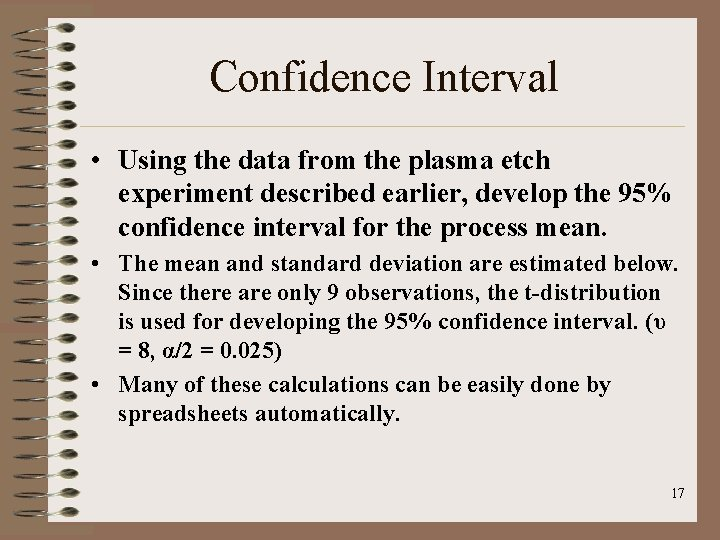Confidence Interval • Using the data from the plasma etch experiment described earlier, develop the 95% confidence interval for the process mean. • The mean and standard deviation are estimated below. Since there are only 9 observations, the t-distribution is used for developing the 95% confidence interval. (υ = 8, α/2 = 0. 025) • Many of these calculations can be easily done by spreadsheets automatically. 17Confidence Interval • Continuing, 18Hypothesis Tests - Review • Hypothesis Tests: – Objective: This section devoted to enabling us to: • • Construct and test a valid statistical hypothesis Conduct comparative statistical tests( t-tests) Relate alpha and beta risk to sample size Conceptually understand analysis of variance (ANOVA) • Interpret the results of various statistical tests: – T-tests, f-tests, chi-square tests. • Understand the foundation for full and fractional factorial • Compute confidence intervals to assess degree of improvements 19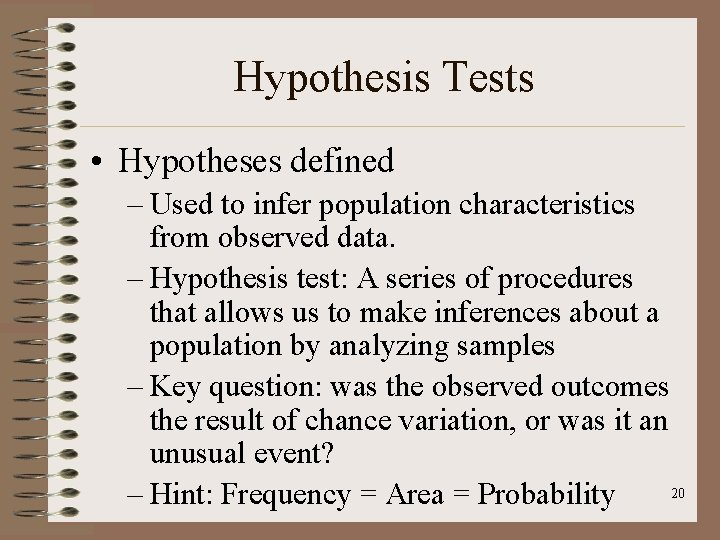Hypothesis Tests • Hypotheses defined – Used to infer population characteristics from observed data. – Hypothesis test: A series of procedures that allows us to make inferences about a population by analyzing samples – Key question: was the observed outcomes the result of chance variation, or was it an unusual event? 20 – Hint: Frequency = Area = Probability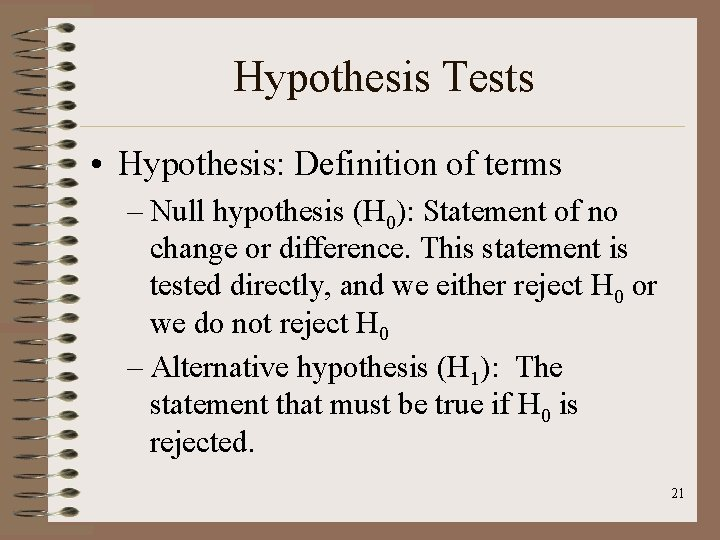Hypothesis Tests • Hypothesis: Definition of terms – Null hypothesis (H 0): Statement of no change or difference. This statement is tested directly, and we either reject H 0 or we do not reject H 0 – Alternative hypothesis (H 1): The statement that must be true if H 0 is rejected. 21Hypothesis Tests • Definition of terms – Type I error: The mistake of rejecting H 0 when it is true. – Type II error: The mistake of failing to reject H 0 when it is false. – alpha risk ( ): Probability of a type I error – beta risk ( ): Probability of a type II error – Test statistic: sample value used in making decision about whether or not to reject H 0 22Hypothesis Tests • Definition of terms – Critical region: Area under the curve corresponding to test statistic that leads to rejection of H 0 – Critical value: The value that separates the critical region from those values that do not lead to rejection of H 0 – Significance level: The probability of rejecting H 0 when it is true – Degrees of freedom: Referred to as d. f. or ν, 23 and = n - 1Hypothesis Tests • Definition of terms – Type I error: Producer’s risk – Type II error: Consumer’s risk – Set so type I is the more serious error type (taking action when none is required) – Levels for and must be established before the test is conducted 24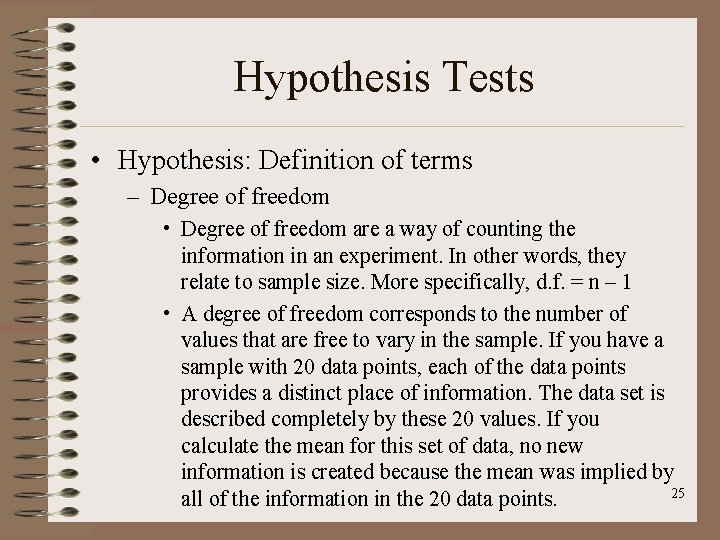Hypothesis Tests • Hypothesis: Definition of terms – Degree of freedom • Degree of freedom are a way of counting the information in an experiment. In other words, they relate to sample size. More specifically, d. f. = n – 1 • A degree of freedom corresponds to the number of values that are free to vary in the sample. If you have a sample with 20 data points, each of the data points provides a distinct place of information. The data set is described completely by these 20 values. If you calculate the mean for this set of data, no new information is created because the mean was implied by 25 all of the information in the 20 data points.Hypothesis Tests • Hypothesis: Definition of terms – Degree of freedom • Once the mean is known, though, all of the information in the data set can be described with any 19 data points. The information in a 20 th data point is now redundant because the 20 th data points has lost the freedom to have any value besides the one imposed on it by the mean • We have one less than the total in our sample because a sample is at least one less than the 26 total population.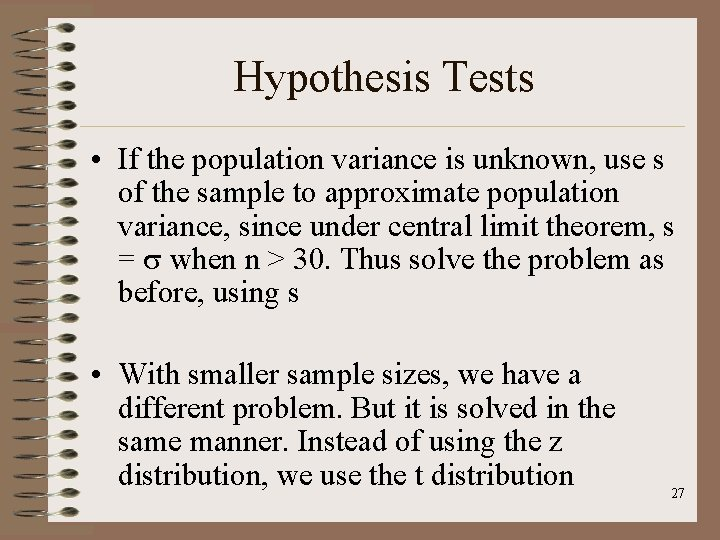Hypothesis Tests • If the population variance is unknown, use s of the sample to approximate population variance, since under central limit theorem, s = when n > 30. Thus solve the problem as before, using s • With smaller sample sizes, we have a different problem. But it is solved in the same manner. Instead of using the z distribution, we use the t distribution 27Hypothesis Tests • Using t distribution when: – Sample is small (<30) – Parent population is essentially normal – Population variance ( ) is unknown – As n decreases, variation within the sample increases, so distribution becomes flatter. 28Hypothesis Tests • Compare the means of two samples: Steps – Understand word problem by writing out null and alternative hypotheses – Select alpha risk level and find critical value – Draw graph of the relation – Insert data into formula – Interpret and conclude 29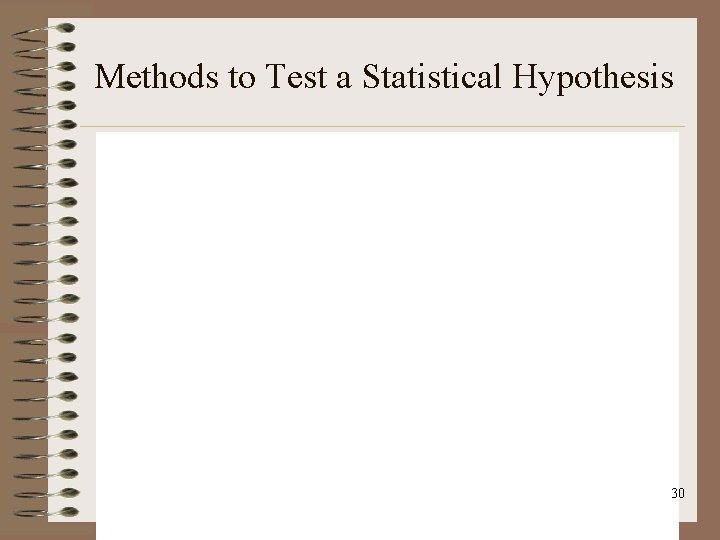Methods to Test a Statistical Hypothesis 30Relationship Between Hypothesis Tests and Confidence Intervals 31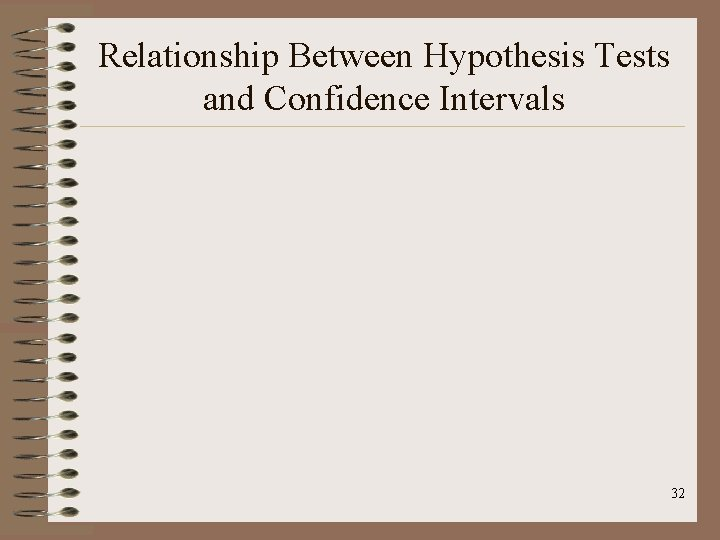Relationship Between Hypothesis Tests and Confidence Intervals 32Relationship between Hypothesis Tests and Confidence Intervals • Using the data from the plasma etch study, can a true process mean of 530 angstroms be expected at a 95% confidence level? • The 95% confidence interval (developed earlier in detail) runs from 555. 85 to 572. 37. Since 530 is not included in this interval, the null hypothesis of μ = 530 is rejected. 33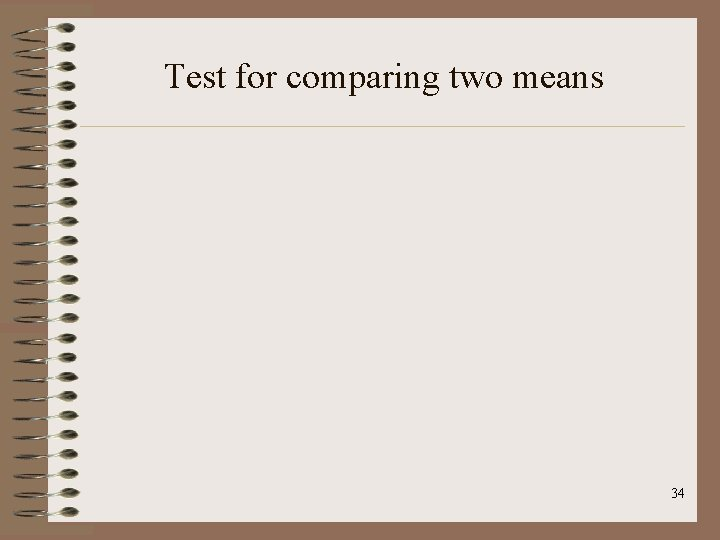Test for comparing two means 34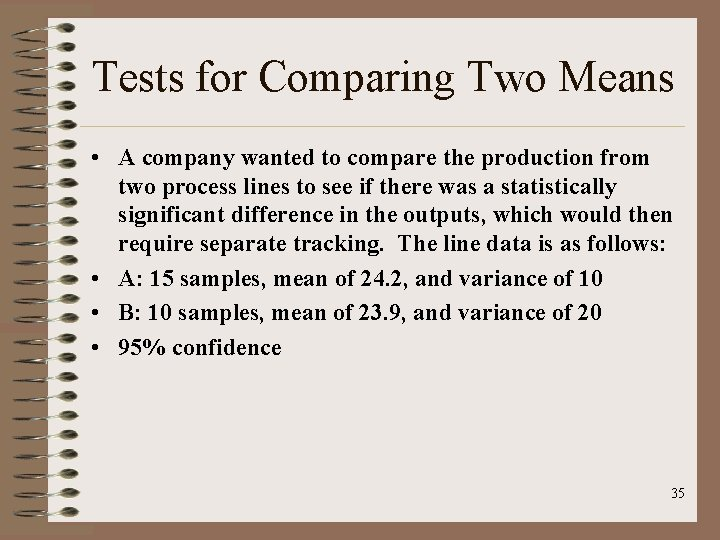Tests for Comparing Two Means • A company wanted to compare the production from two process lines to see if there was a statistically significant difference in the outputs, which would then require separate tracking. The line data is as follows: • A: 15 samples, mean of 24. 2, and variance of 10 • B: 10 samples, mean of 23. 9, and variance of 20 • 95% confidence 35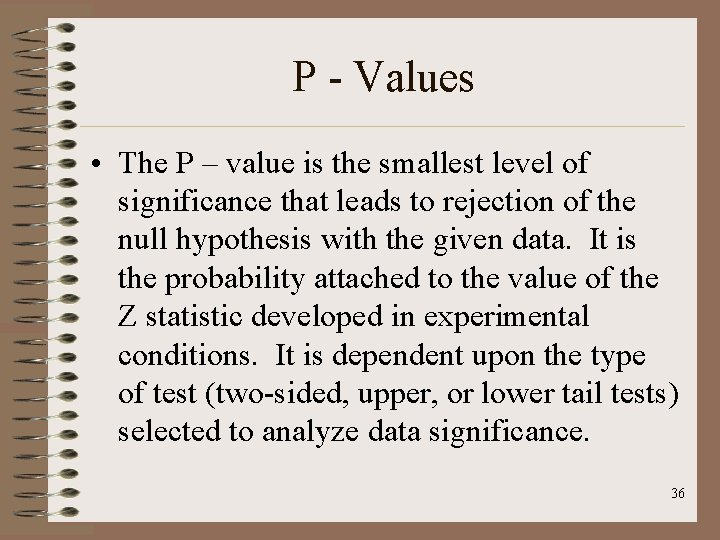P - Values • The P – value is the smallest level of significance that leads to rejection of the null hypothesis with the given data. It is the probability attached to the value of the Z statistic developed in experimental conditions. It is dependent upon the type of test (two-sided, upper, or lower tail tests) selected to analyze data significance. 36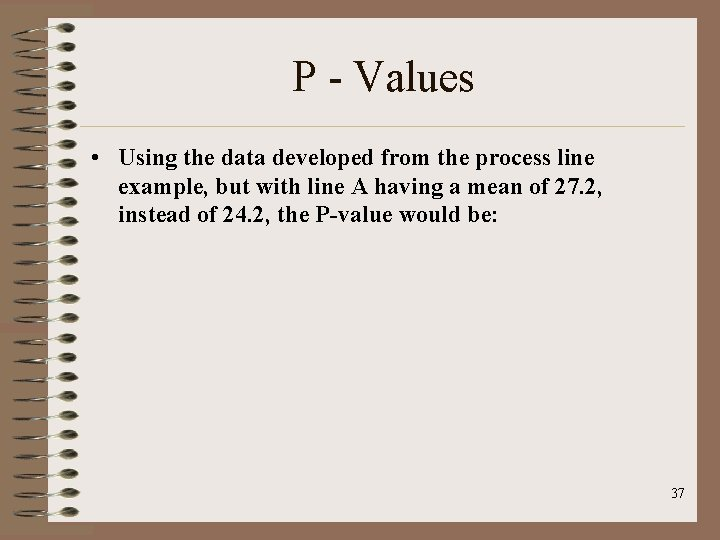P - Values • Using the data developed from the process line example, but with line A having a mean of 27. 2, instead of 24. 2, the P-value would be: 37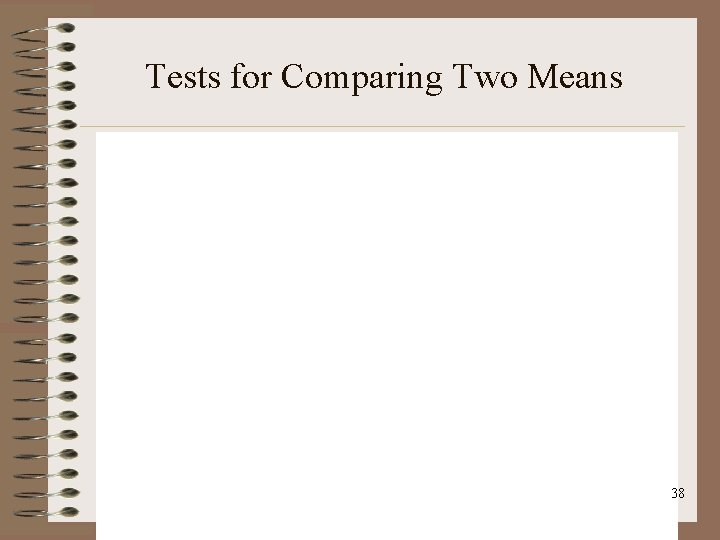Tests for Comparing Two Means 38Tests for Comparing Two Means 39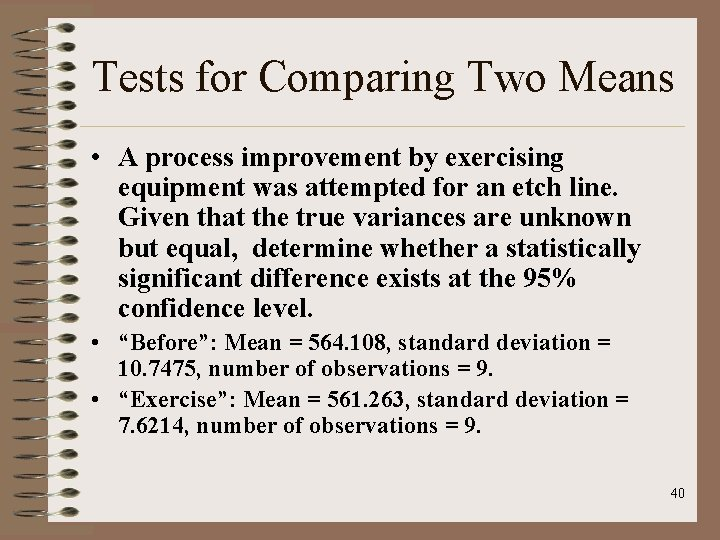Tests for Comparing Two Means • A process improvement by exercising equipment was attempted for an etch line. Given that the true variances are unknown but equal, determine whether a statistically significant difference exists at the 95% confidence level. • “Before”: Mean = 564. 108, standard deviation = 10. 7475, number of observations = 9. • “Exercise”: Mean = 561. 263, standard deviation = 7. 6214, number of observations = 9. 40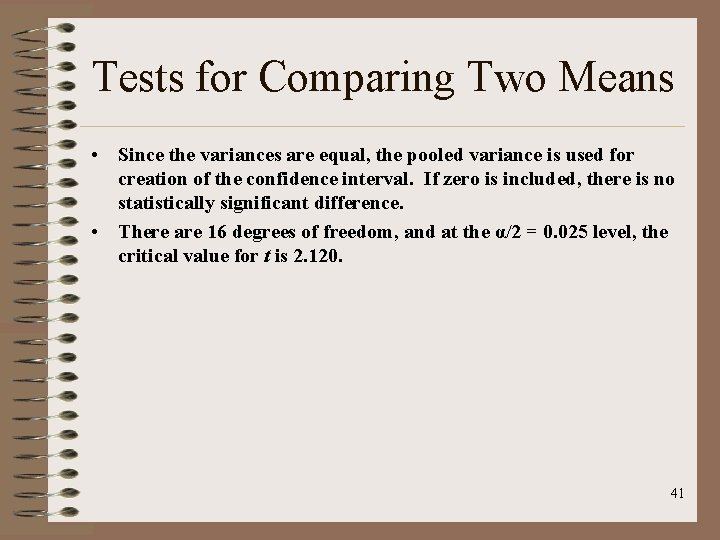Tests for Comparing Two Means • Since the variances are equal, the pooled variance is used for creation of the confidence interval. If zero is included, there is no statistically significant difference. • There are 16 degrees of freedom, and at the α/2 = 0. 025 level, the critical value for t is 2. 120. 41Test for comparing two means 42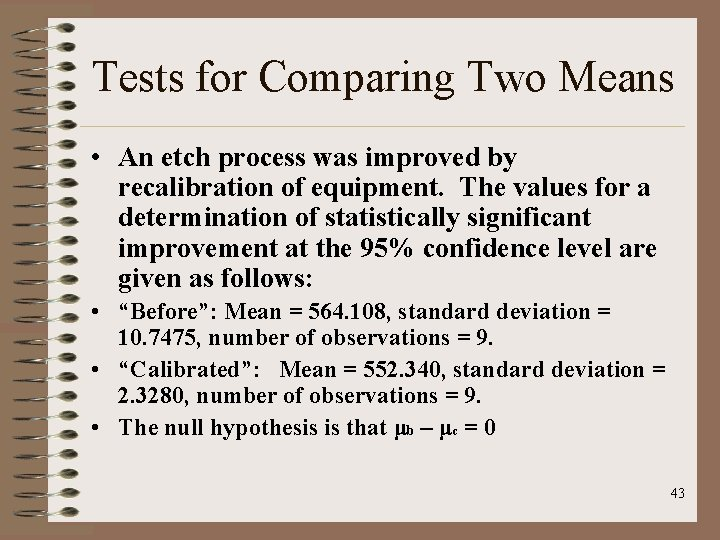Tests for Comparing Two Means • An etch process was improved by recalibration of equipment. The values for a determination of statistically significant improvement at the 95% confidence level are given as follows: • “Before”: Mean = 564. 108, standard deviation = 10. 7475, number of observations = 9. • “Calibrated”: Mean = 552. 340, standard deviation = 2. 3280, number of observations = 9. • The null hypothesis is that μb – μc = 0 43Tests for Comparing Two Means • The first task is to determine the number of degrees of freedom and the appropriate critical value. 44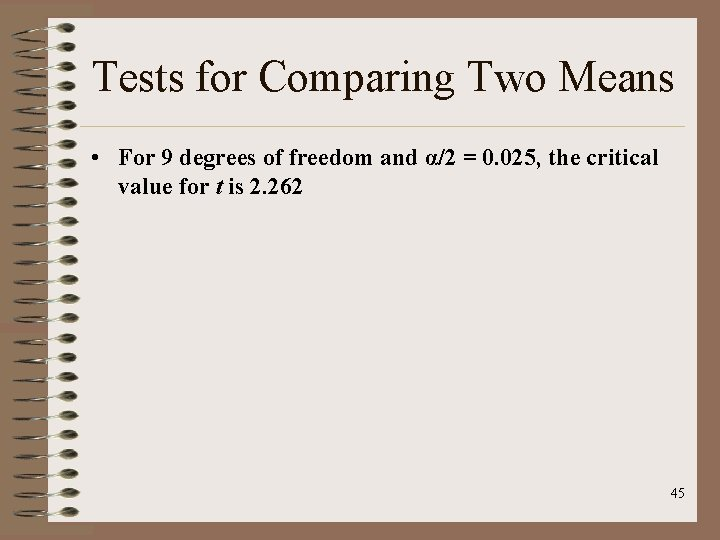Tests for Comparing Two Means • For 9 degrees of freedom and α/2 = 0. 025, the critical value for t is 2. 262 45Tests for Comparing Two Means 46Tests for Comparing Two Means • Two materials were compared in a wear test as a paired, randomized experiment. The coded data are tabulated below, as well as the differences for each pairing. Run 1 2 3 4 5 6 7 8 9 10 A 13. 2 8. 2 10. 9 14. 3 10. 7 6. 6 9. 5 10. 8 8. 8 13. 3 B 14. 0 8. 8 11. 2 14. 2 11. 8 6. 4 9. 8 11. 3 9. 3 13. 6 Δ 0. 8 0. 6 0. 3 -0. 1 1. 1 -0. 2 0. 3 0. 5 0. 3 47Tests for Comparing Two Means • The mean, standard deviation, and 95% confidence intervals are constructed below, with nine degrees of freedom. If the interval does not contain zero, the null hypothesis of “no difference” is rejected. 48Test for Comparing Two Variances • The variances can also be used to determine the likelihood of observations being part of the same population. For variances, the test used is the F-test since the ratio of variances will follow the Fdistribution. This test is also the basic test used in the ANOVA method covered in the next section. 49F - Test • The F distribution is developed from three parameters: α (level of confidence), and the two degrees of freedom for the variances under comparison. The null hypothesis is typically one where the variances are equal, which would yield an allowed set of values that F can be and still not reject the null hypothesis. 50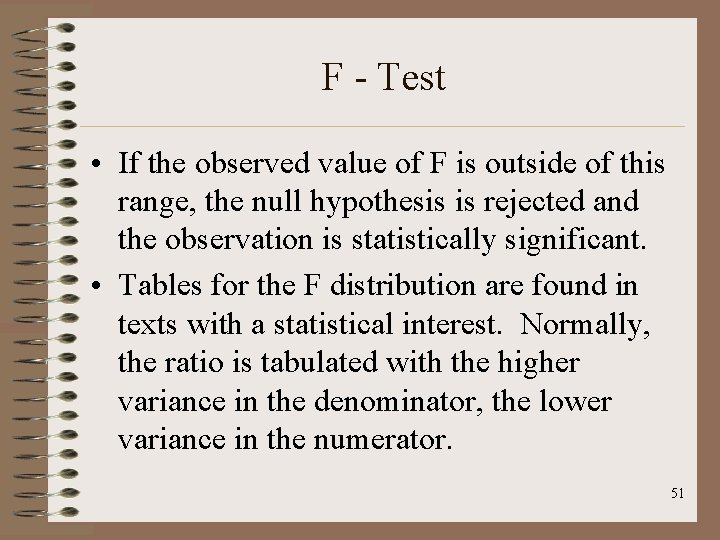F - Test • If the observed value of F is outside of this range, the null hypothesis is rejected and the observation is statistically significant. • Tables for the F distribution are found in texts with a statistical interest. Normally, the ratio is tabulated with the higher variance in the denominator, the lower variance in the numerator. 51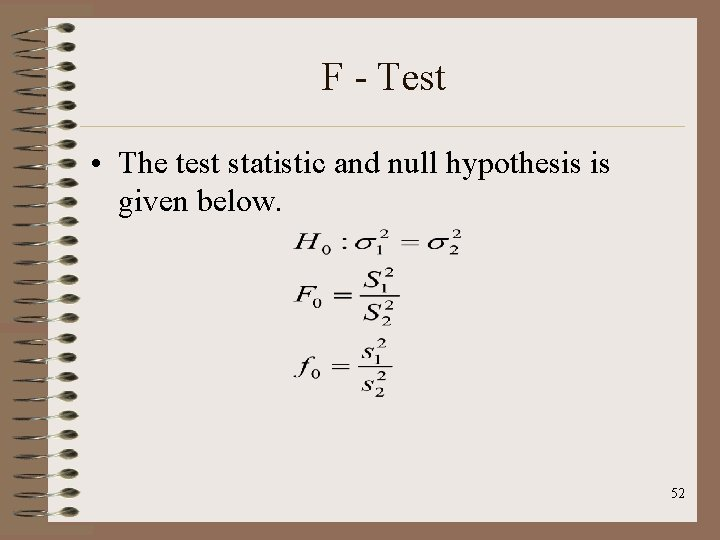F - Test • The test statistic and null hypothesis is given below. 52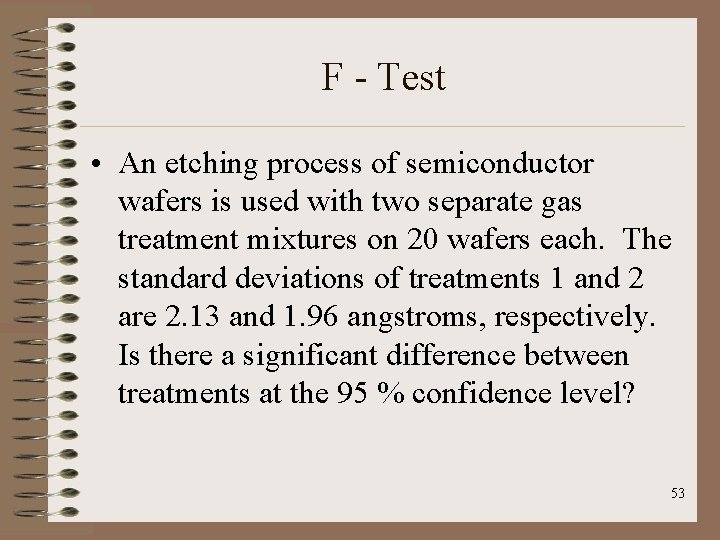F - Test • An etching process of semiconductor wafers is used with two separate gas treatment mixtures on 20 wafers each. The standard deviations of treatments 1 and 2 are 2. 13 and 1. 96 angstroms, respectively. Is there a significant difference between treatments at the 95 % confidence level? 53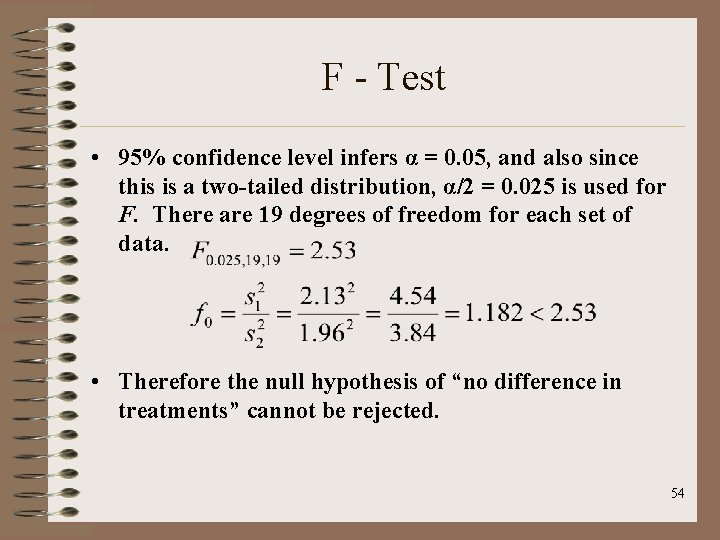F - Test • 95% confidence level infers α = 0. 05, and also since this is a two-tailed distribution, α/2 = 0. 025 is used for F. There are 19 degrees of freedom for each set of data. • Therefore the null hypothesis of “no difference in treatments” cannot be rejected. 54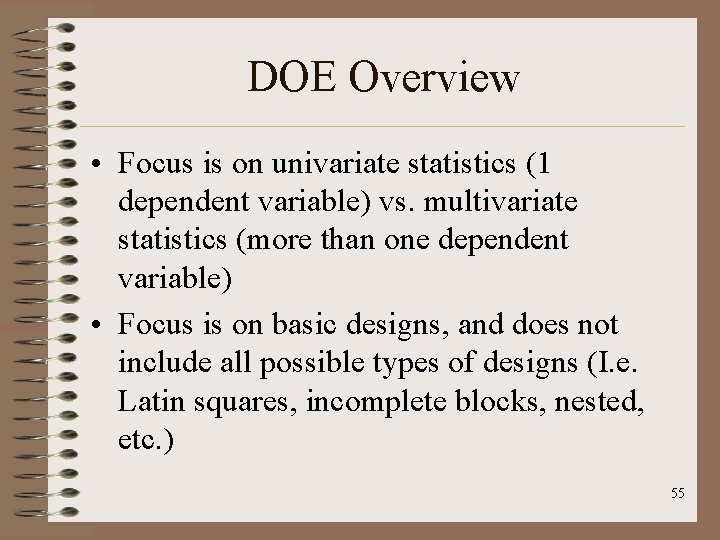DOE Overview • Focus is on univariate statistics (1 dependent variable) vs. multivariate statistics (more than one dependent variable) • Focus is on basic designs, and does not include all possible types of designs (I. e. Latin squares, incomplete blocks, nested, etc. ) 55DOE Overview • One key item to keep in clear focus while performing a designed experiment: • Why are we doing it? • According to Taguchi (among others) it is to refine our process to yield one of the following quality outcomes: 56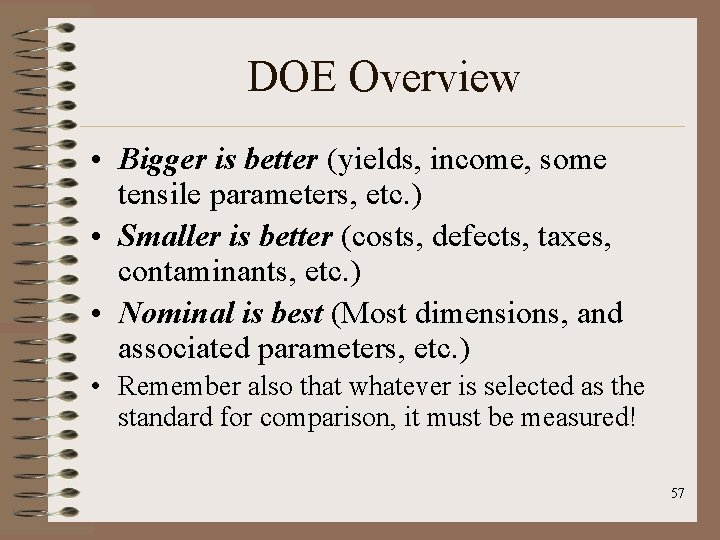DOE Overview • Bigger is better (yields, income, some tensile parameters, etc. ) • Smaller is better (costs, defects, taxes, contaminants, etc. ) • Nominal is best (Most dimensions, and associated parameters, etc. ) • Remember also that whatever is selected as the standard for comparison, it must be measured! 57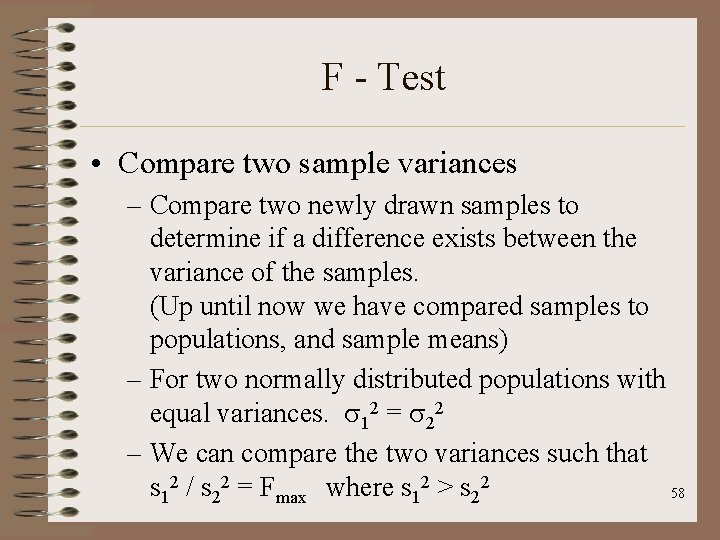F - Test • Compare two sample variances – Compare two newly drawn samples to determine if a difference exists between the variance of the samples. (Up until now we have compared samples to populations, and sample means) – For two normally distributed populations with equal variances. 12 = 22 – We can compare the two variances such that s 12 / s 22 = Fmax where s 12 > s 22 58F - Test • Compare two sample variances – F tests for equality of the variances and uses the f-distribution. – This works just like method used with the t distribution: critical value is compared to test statistics. – If two variances are equal, F = s 12 / s 22 = 1 , thus we compare ratios of variances. 59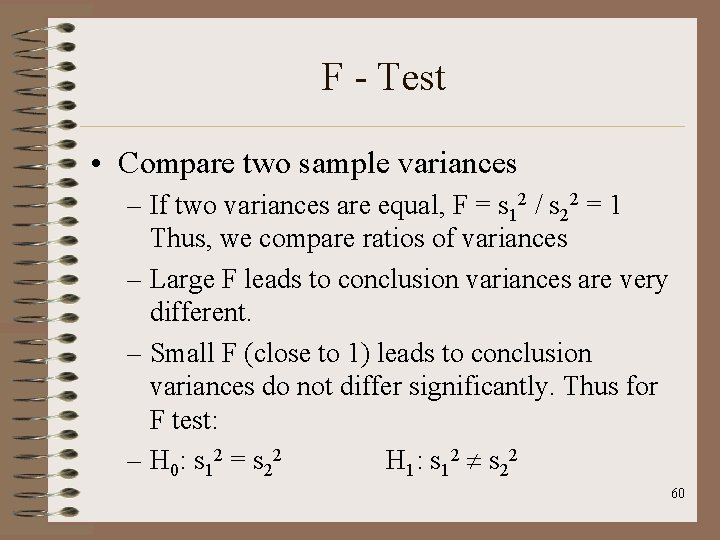F - Test • Compare two sample variances – If two variances are equal, F = s 12 / s 22 = 1 Thus, we compare ratios of variances – Large F leads to conclusion variances are very different. – Small F (close to 1) leads to conclusion variances do not differ significantly. Thus for F test: – H 0: s 12 = s 22 H 1: s 12 s 22 60F - Test • Compare two sample variances – F tables • Several exist, depending on alpha level. – Using F tables requires 3 bits of information. • Chosen alpha risk • Degree of freedom (n 1 – 1) for numerator term. • Degree of freedom (n 2 – 1) for denominator term. 61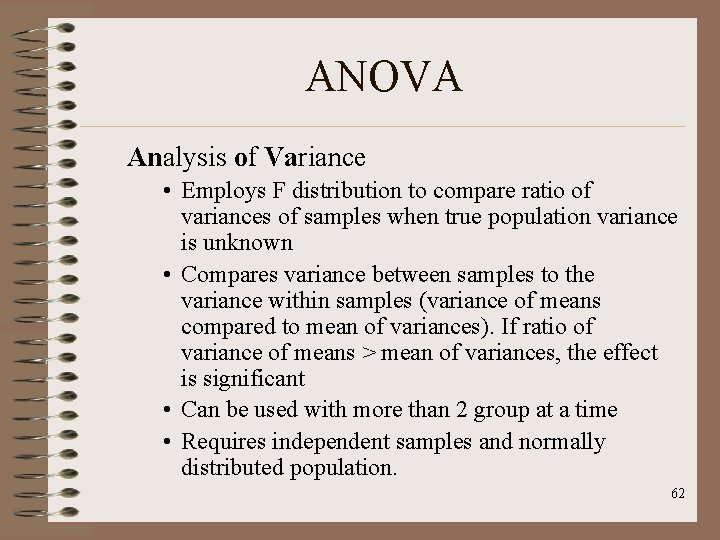ANOVA Analysis of Variance • Employs F distribution to compare ratio of variances of samples when true population variance is unknown • Compares variance between samples to the variance within samples (variance of means compared to mean of variances). If ratio of variance of means > mean of variances, the effect is significant • Can be used with more than 2 group at a time • Requires independent samples and normally distributed population. 62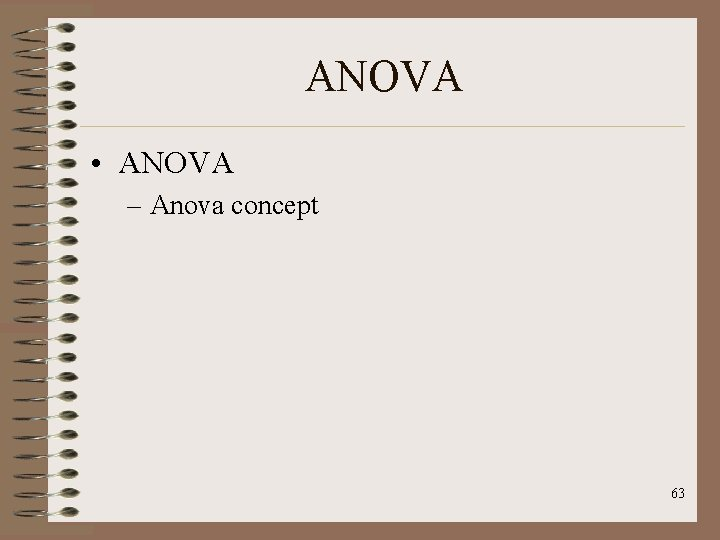ANOVA • ANOVA – Anova concept 63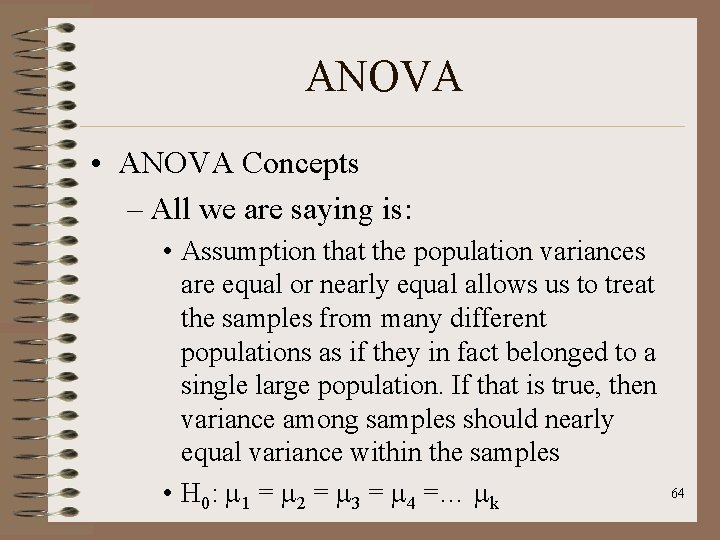ANOVA • ANOVA Concepts – All we are saying is: • Assumption that the population variances are equal or nearly equal allows us to treat the samples from many different populations as if they in fact belonged to a single large population. If that is true, then variance among samples should nearly equal variance within the samples • H 0: 1 = 2 = 3 = 4 =… k 64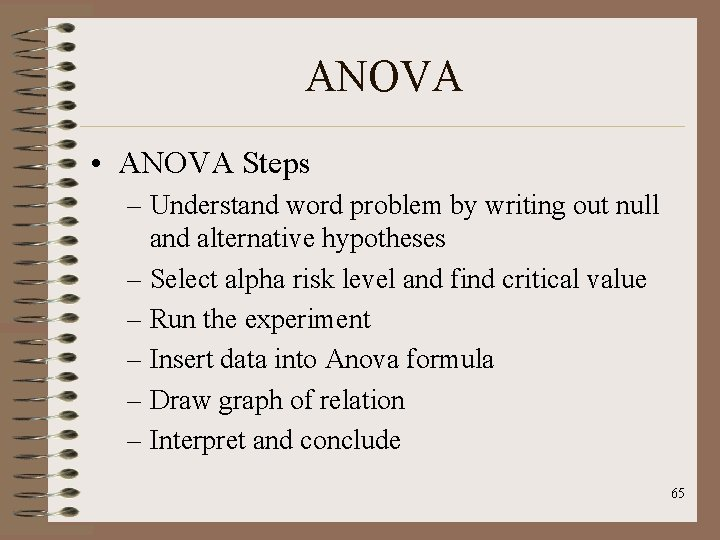ANOVA • ANOVA Steps – Understand word problem by writing out null and alternative hypotheses – Select alpha risk level and find critical value – Run the experiment – Insert data into Anova formula – Draw graph of relation – Interpret and conclude 65ANOVA • Analysis of Variance (ANOVA) is a powerful tool for determining significant contributors towards process responses. The process is a vector decomposition of a response, and can be modified for a wide variety of models. • Can be used with more than 2 groups at a time • Requires independent, normally distributed samples. 66ANOVA • ANOVA decomposition is an orthogonal vector breakdown of a response (which is why independence is required), so for a process with factors A and B, as tabulated below: 67ANOVA • The ANOVA values are given by: 68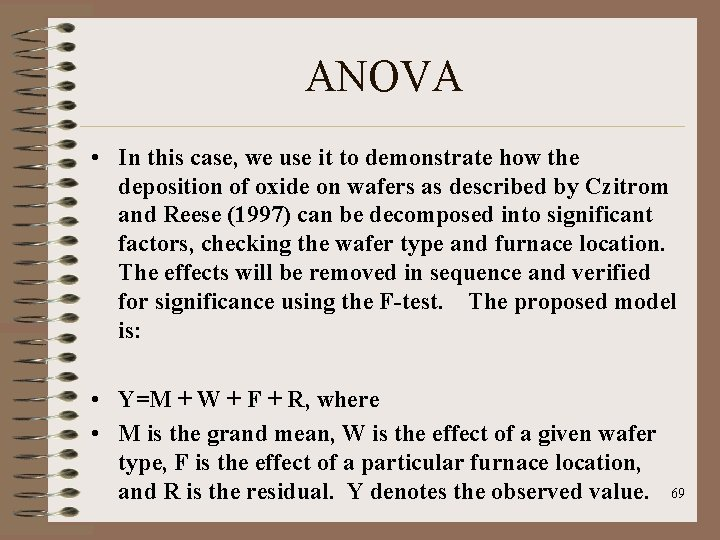ANOVA • In this case, we use it to demonstrate how the deposition of oxide on wafers as described by Czitrom and Reese (1997) can be decomposed into significant factors, checking the wafer type and furnace location. The effects will be removed in sequence and verified for significance using the F-test. The proposed model is: • Y=M + W + F + R, where • M is the grand mean, W is the effect of a given wafer type, F is the effect of a particular furnace location, and R is the residual. Y denotes the observed value. 69ANOVA • F-test in ANOVA • The estimator for a given variance in ANOVA is the mean sum of squares (MS). For a given factor, its MS can be calculated as noted before, and the ratio of the factor MS and residual MS compared to the Fdistribution for significance. To do so, the level of significance must be defined to establish the Type I error limit. 70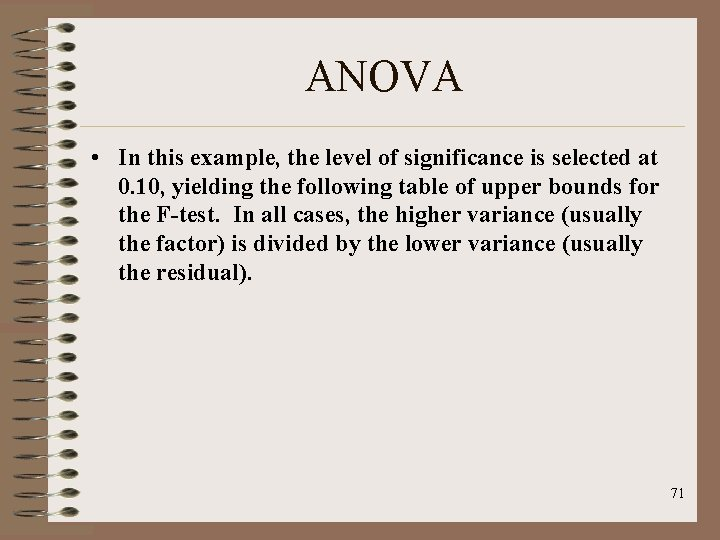ANOVA • In this example, the level of significance is selected at 0. 10, yielding the following table of upper bounds for the F-test. In all cases, the higher variance (usually the factor) is divided by the lower variance (usually the residual). 71ANOVA • The set of means observed in the process, broken down by wafer type and furnace location are (in mils = 0. 001 inch) tabulated below. The grand mean is 92. 1485. 72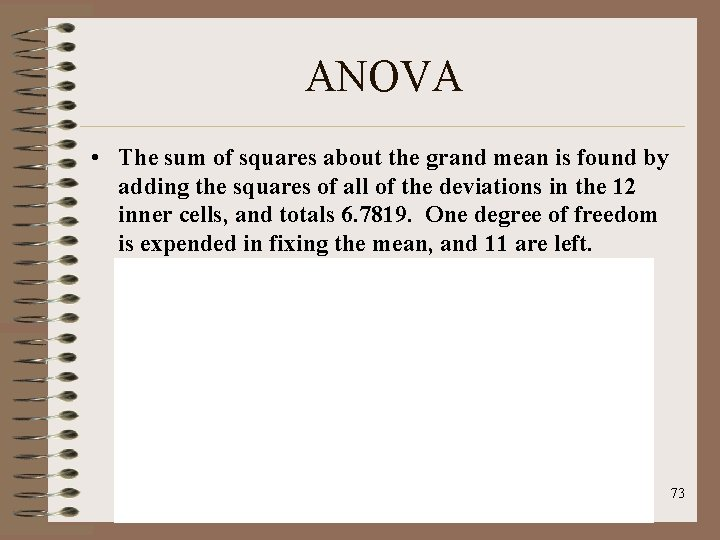ANOVA • The sum of squares about the grand mean is found by adding the squares of all of the deviations in the 12 inner cells, and totals 6. 7819. One degree of freedom is expended in fixing the mean, and 11 are left. 73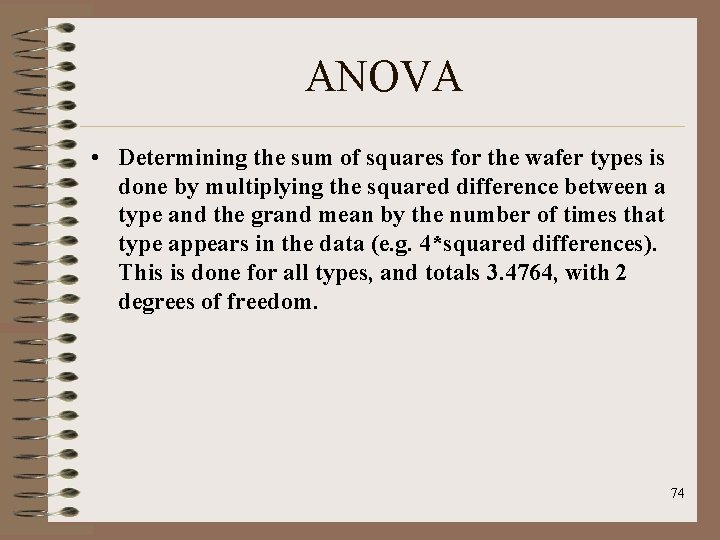ANOVA • Determining the sum of squares for the wafer types is done by multiplying the squared difference between a type and the grand mean by the number of times that type appears in the data (e. g. 4*squared differences). This is done for all types, and totals 3. 4764, with 2 degrees of freedom. 74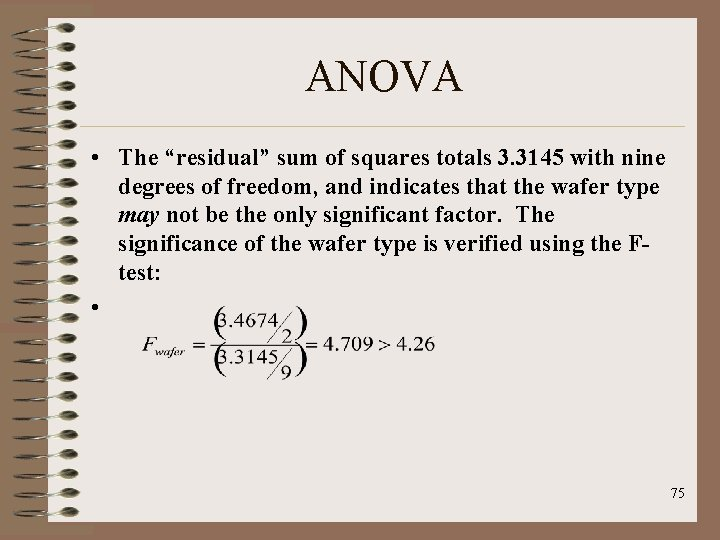ANOVA • The “residual” sum of squares totals 3. 3145 with nine degrees of freedom, and indicates that the wafer type may not be the only significant factor. The significance of the wafer type is verified using the Ftest: • 75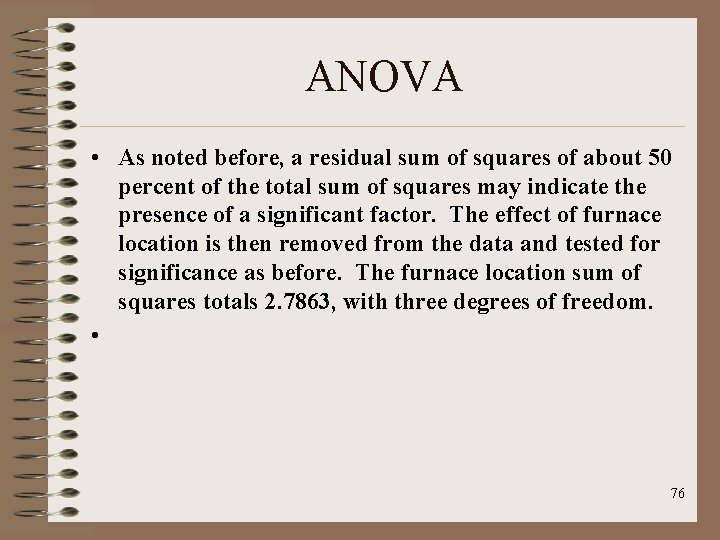ANOVA • As noted before, a residual sum of squares of about 50 percent of the total sum of squares may indicate the presence of a significant factor. The effect of furnace location is then removed from the data and tested for significance as before. The furnace location sum of squares totals 2. 7863, with three degrees of freedom. • 76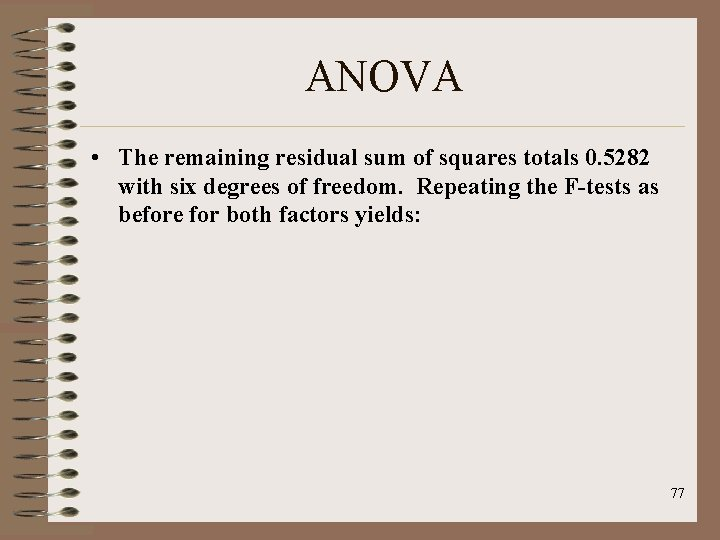ANOVA • The remaining residual sum of squares totals 0. 5282 with six degrees of freedom. Repeating the F-tests as before for both factors yields: 77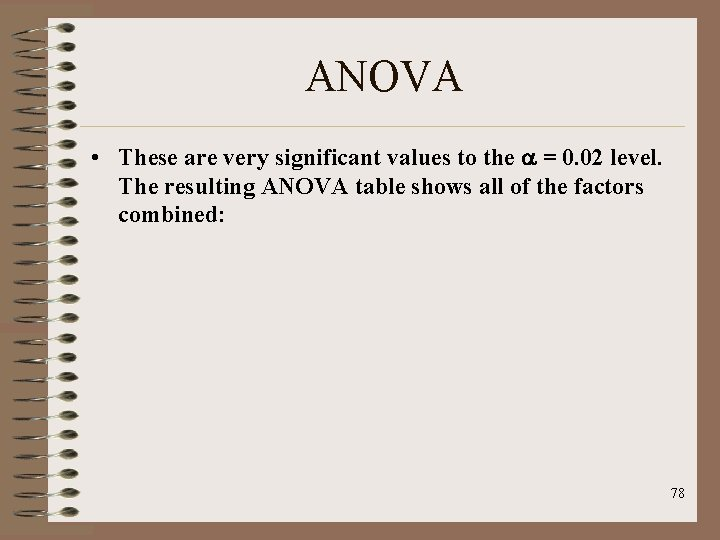ANOVA • These are very significant values to the = 0. 02 level. The resulting ANOVA table shows all of the factors combined: 78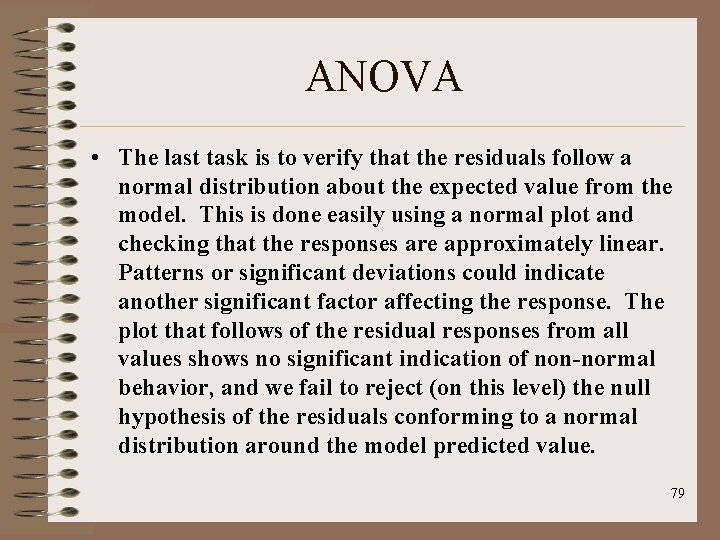ANOVA • The last task is to verify that the residuals follow a normal distribution about the expected value from the model. This is done easily using a normal plot and checking that the responses are approximately linear. Patterns or significant deviations could indicate another significant factor affecting the response. The plot that follows of the residual responses from all values shows no significant indication of non-normal behavior, and we fail to reject (on this level) the null hypothesis of the residuals conforming to a normal distribution around the model predicted value. 79ANOVA 80